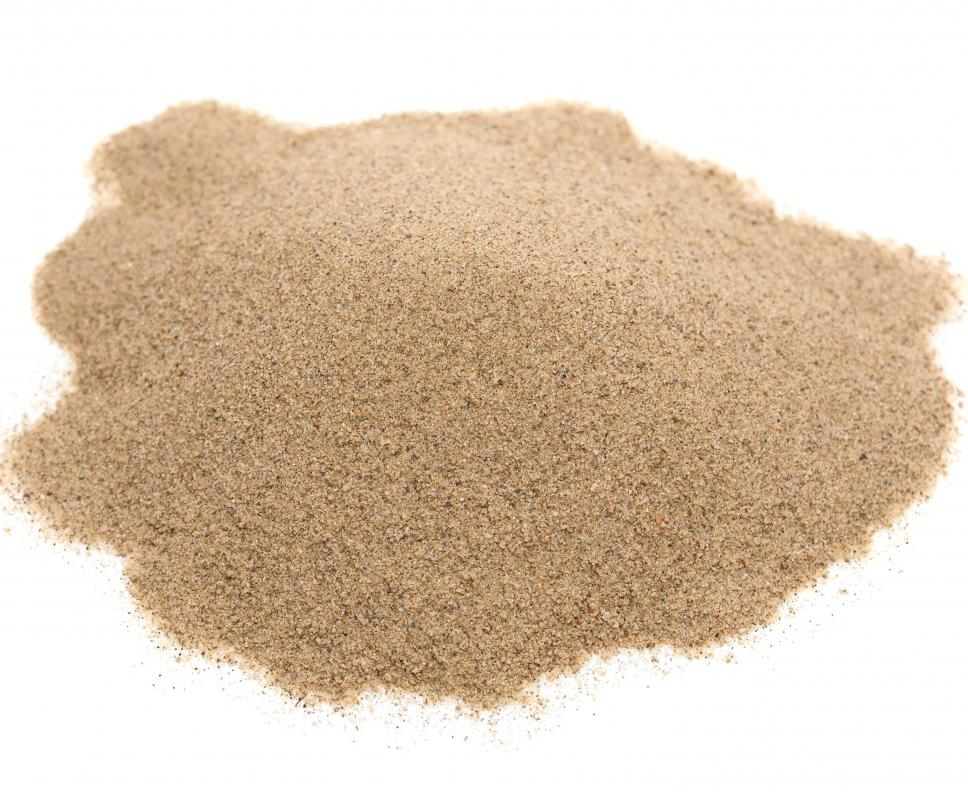# What Is a Formula Unit?

Richard Nelson

Atoms of different elements can join together in two main ways. In an ionic bond, a metal gives one or more electrons to a non-metal, forming oppositely charged ions that are held together by electrical attraction. Covalent bonds form by two or more non-metals sharing electrons. Ionic compounds do not form molecules as such, but instead, in the solid form, consist of three-dimensional crystal lattices containing large numbers of atoms. Some covalent compounds can form similar crystal networks. A formula unit is the smallest ratio between atoms of different elements in this kind of structure that can be expressed as whole numbers.

#### Ionic Compounds

A simple example is sodium chloride, or common salt, a compound of the elements sodium and chlorine. A salt crystal consists of positively charged sodium ions bonded to negatively charged chloride ions — the negative ions formed by non-metals end in “–ide.” The crystal contains enormous numbers of sodium and chloride ions, but there is one chloride ion for every sodium ion, so the formula unit shows one of each. The chemical symbols for sodium and chlorine are Na and Cl, respectively, so the formula unit is written as NaCl.The formula unit for the crystalline quartz that makes up sand is SiO2.

Many ionic compounds are slightly more complex, for example, aluminum oxide. Here, oxygen is looking for two electrons, and aluminum wants to give three. They can therefore form a stable compound with the formula unit Al2O3. The number of atoms of an element in any type of chemical formula appears subscripted and to the right of the symbol for that element. If there is only one atom present, the subscripted number is omitted.

#### Covalent Compounds

Although covalent compounds and substances often form distinct, self-contained molecules, they can also form crystalline structures. For example, silicon dioxide, also known as silica, can form crystals. These are commonly known as quartz, and, like salt, consist of huge numbers of two different atoms — in this case, silicon and oxygen — but held together by covalent bonds. Since the ratio of oxygen to silicon atoms is 2:1, quartz has the formula unit SiO2.

#### Related Terms

There are a couple of other, related, terms that might cause some confusion. Empirical formula is a more general term for the simplest ratio of elements in a compound, whether ionic or covalent, crystalline or not. In a crystalline compound, it is the same as the formula unit, but the term also applies to self-contained, non-crystalline covalent molecules. The molecular formula is the actual number of atoms of each element in a self-contained covalent molecule, and does not apply to ionic compounds, as these do not form distinct molecules.

In ionic compounds, formula unit tends to be used to show the simplest ratio of atoms, while in non-crystalline covalent compounds, the usual term for this is the empirical formula. For example, the carbon-hydrogen compounds acetylene and benzene both contain the same number of carbon as hydrogen atoms, and therefore both have the empirical formula CH. The molecular formula for acetylene, however, is C2H2, while that for benzene is C6H6. These are very different compounds, with different properties.

In many covalent compounds, the empirical formula and the molecular formula are the same. In water, for example, they are both H2O. This, however, is seldom the case with organic compounds, which may be very complex. In these compounds, there is often more than one possibility for the same ratio of elements, as already noted with acetylene and benzene. Sometimes there are many different variations.

In many cases, even the molecular formula does not tell the whole story. For example, glucose and fructose, two different kinds of sugar, have the same molecular formula, C6H12O6. The hydrogen and oxygen atoms are, however, arranged slightly differently, giving the two compounds slightly different properties. The empirical formula for glucose and fructose is CH2O.

#### Determining the Formula Unit for a Compound

In many cases, all that is required to find the formula unit for an ionic compound, and for some simple crystalline covalent compounds, is knowledge of how many single bonds the elements are able to form. In the case of metals, this is the number of electrons they can provide, while in non-metals this is the number of electrons they can accept or, in the case of covalent compounds, share. This is known as the oxidation number. It is normally positive in metals — which lose negatively charged electrons in forming compounds — and negative in non-metals — which gain electrons, at least when combining with metals.

Returning to the aluminum oxide example, aluminum has an oxidation number of +3, while oxygen has an oxidation number of -2. To find the formula unit for aluminum oxide, these numbers are simply swapped round to give a compound with three atoms of oxygen for every two of aluminum: Al2O3. The same procedure works for many other ionic compounds, and for some simple covalent compounds. There are, however, complications, because some elements can have more than one oxidation number, depending on circumstances. Iron, for example, can be +2 or +3, and many non-metals can have multiple oxidation numbers, which may be positive in some covalent compounds.

## You might also Like